# Symplectic structure

(diff) ← Older revision | Latest revision (diff) | Newer revision → (diff)
An infinitesimal structure of order one on an even-dimensional smooth orientable manifoldwhich is defined by a non-degenerate-formon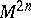. Every tangent space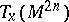has the structure of a symplectic space with skew-symmetric scalar product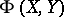. All frames tangent toadapted to the symplectic structure (that is, frames with respect to whichhas the canonical form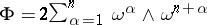) form a principal fibre bundle overwhose structure group is the symplectic group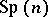. Specifying a symplectic structure onis equivalent to specifying an-structure on(cf.-structure).
Given a symplectic structure on, there is an isomorphism between the modules of vector fields and-forms on, under which a vector fieldis associated with a-form,. In this context, the image of the Lie bracketis called the Poisson bracket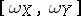. In particular, whenandare exact differentials, one obtains the concept of the Poisson bracket of two functions on, which generalizes the corresponding classical concept.
A symplectic structure is also called an almost-Hamiltonian structure, and ifis closed, i.e.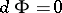, a Hamiltonian structure, though the conditionis sometimes included in the definition of a symplectic structure. These structures find application in global analytical mechanics, since the cotangent bundle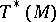of any smooth manifoldadmits a canonical Hamiltonian structure. It is defined by the form, where the-formon, called the Liouville form, is given by:for any tangent vectorat the point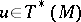, whereis the projection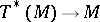. If one chooses local coordinates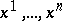on, and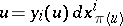, then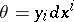, so that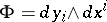. In classical mechanicsis interpreted as the configuration space andas the phase space.
A vector fieldon a manifoldwith a Hamiltonian structure is called a Hamiltonian vector field (or a Hamiltonian system) if the-form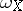is closed. If, in addition, it is exact, that is,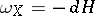, thenis called a Hamiltonian onand is a generalization of the corresponding classical concept.